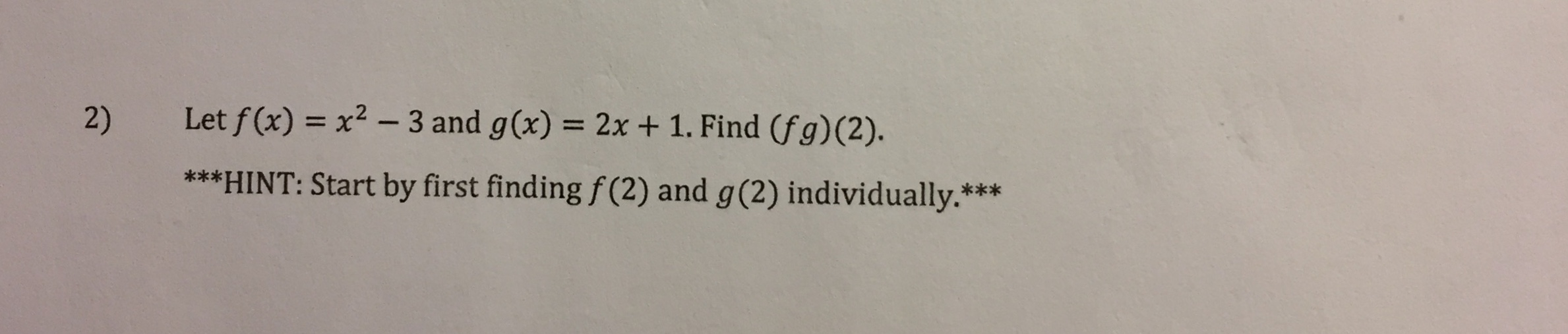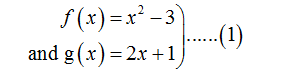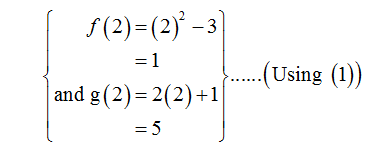# Let f (x) = x2 - 3 and g(x) = 2x + 1. Find (fg)(2).2)***HINT: Start by first finding f (2) and g(2) individually.***

Question
1 viewshelp_outlineImage TranscriptioncloseLet f (x) = x2 - 3 and g(x) = 2x + 1. Find (fg)(2). 2) ***HINT: Start by first finding f (2) and g(2) individually.*** fullscreen
check_circle

Step 1

The given functions are,Step 2

To find the value of (fg)(2).

Step 3

Evaluating values for f(2) and g(2) respectively,...

### Want to see the full answer?

See Solution

#### Want to see this answer and more?

Solutions are written by subject experts who are available 24/7. Questions are typically answered within 1 hour.*

See Solution
*Response times may vary by subject and question.
Tagged in

### Algebra